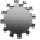Conference TopicsContributions related, but not limited, to the following topics of interest are welcome:

Theory of Statistics
Statistical Inference
Linear Models and GLMs
Bayesian Methods
Decision Theory
Nonparametric Methods
Fuzzy Statistics
Applied Statistics
Sampling
Regression
Time Series
Experimental Design
Computational Statistics
Spatial Statistics
Data Mining
Statistical Quality Control
Statistical Simulation
Categorical Data Analysis
Statistical Modeling
Multivariate Methods
Probability Theory
Stochastic Processes
Stochastic Systems
Distribution Theory
Order Statistics & Record values
Biostatistics
Small Area Estimation
Sampling Design
Population Statistics
Statistics Education
Actuarial – Insurance Mathematics
Bayesian Statistics
Biostatistics – Biometry – Medical Statistics
Computational  Statistics
Data Analysis
Decision Theory
Econometrics
Educational Statistics
Environmetrics
Financial Statistics
Mathematical Statistics
Operations Research
Probability Theory
Reliability
Risk Management
Social Statistics
Stochastic Processes
Time Series - Forecasting